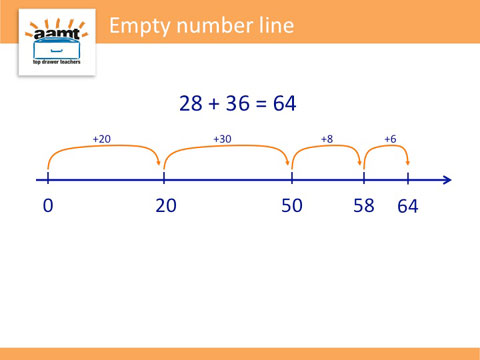Place value

The structure of the place value system, and the ways in which numbers can be partitioned, assist students to develop flexibility.

For example, to solve 9 + 7 one can think of building to 10 by splitting the 7 into 6 and 1, and treating it as 9 + 1 + 6.

This is helpful as 10 + 6 is easier to solve than 9 + 7.

To solve 28 + 36 mentally, one easy way is to add 20 and 30, then 8 and 6, knowing that 8 and 6 add to 14.

Use of an empty number line can make students' thinking visible and represent different strategies used.For example, to multiply 36 by 5, we can split 36 into 30 and 6 and multiply each by 5. Add the partial products together: (30 $$\times$$ 5) + (6 $$\times$$ 5) so 150 + 30 = 180.